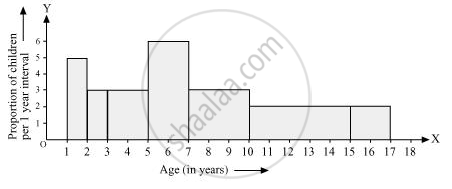# A Random Survey of the Number of Children of Various Age Groups Playing in a Park Was Found as Follows - Mathematics

A random survey of the number of children of various age groups playing in a park was found as follows:-

 Age (in years) Number of children 1 - 2 5 2 - 3 3 3 - 5 6 5 - 7 12 7 - 10 9 10 - 15 10 15 - 17 4

Draw a histogram to represent the data above.

#### Solution

Here, it can be observed that the data has class intervals of varying width. The proportion of children per 1 year interval can be calculated as follows.

 Age (in years) Frequency (Number of children) Width of class Length of rectangle 1 − 2 5 1 (5 x 1)/1=5 2 − 3 3 1 (3 x 1)/1=3 3 − 5 6 2 (6 x 1)/2=3 5 − 7 12 2 (12 x 1)/2=6 7 − 10 9 3 (9 x 1)/3=6 10 − 15 10 5 (10 x 1)/5=2 15 − 17 4 2 (4 x 1)/2=2

Taking the age of children on x-axis and proportion of children per 1 year interval on y-axis, the histogram can be drawn as follows.Concept: Graphical Representation of Data
Is there an error in this question or solution?

#### APPEARS IN

NCERT Class 9 Maths
Chapter 14 Statistics
Exercise 14.3 | Q 8 | Page 261